• +91 9971497814
• info@interviewmaterial.com

# Chapter 7 Triangles Ex-7.2 Interview Questions Answers

### Related Subjects

Question 1 :

In an isosceles triangle ABC, with AB = AC, the bisectorsof B and C intersect each other at O. Join A to O.Show that:

(i) OB = OC (ii) AO bisects A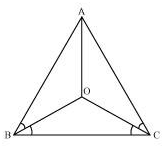(i) It is given that in triangle ABC, AB = AC

ACB = ABC (Angles opposite to equal sides of atriangle are equal)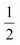ACB =ABC

OCB = OBC

OB = OC (Sides opposite to equal angles of a triangle arealso equal)

(ii) In ΔOAB and ΔOAC,

AO =AO (Common)

AB = AC (Given)

OB = OC (Proved above)

Therefore, ΔOAB ΔOAC (BySSS congruence rule)

BAO = CAO (CPCT)

AO bisects A.

Question 2 :

In ΔABC, AD is the perpendicular bisector of BC (see thegiven figure). Show that ΔABC is an isosceles triangle in which AB = AC.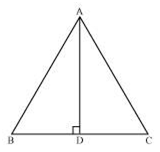CD = BD (AD is the perpendicular bisector of BC)

AB = AC (By CPCT)

Therefore, ABC is an isosceles triangle inwhich AB = AC.

Question 3 :

ABC is an isosceles triangle in which altitudes BE and CFare drawn to equal sides AC and AB respectively (see the given figure). Showthat these altitudes are equal.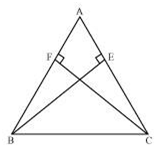In ΔAEB and ΔAFC,

AEB and AFC (Each 90º)

A = A (Common angle)

AB = AC (Given)

ΔAEB ΔAFC (By AAS congruence rule)

BE = CF (By CPCT)

Question 4 :

ABC is a triangle in which altitudes BE and CF to sides ACand AB are equal (see the given figure). Show that

(i)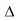ABEACF

(ii) AB = AC, i.e., ABC is an isosceles triangle.(i) In ΔABE and ΔACF,

AEB = AFC (Each90º)

A = A (Common angle)

BE = CF (Given)

ΔABE ΔACF (By AAS congruence rule)

(ii) It has already been proved that

ΔABE ΔACF

AB = AC (By CPCT)

Question 5 :

ABC and DBC are two isosceles triangles on the same baseBC (see the given figure). Show that ABD = ACD.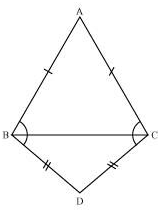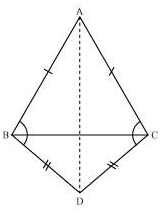In ΔABD and ΔACD,

AB = AC (Given)

BD = CD (Given)

ΔABD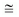ΔACD (By SSS congruence rule)

ABD = ACD (By CPCT)

Question 6 :

ΔABC is an isosceles triangle in which AB = AC. Side BA isproduced to D such that AD = AB (see the given figure). Show that BCD is a right angle.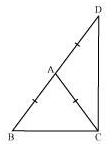In ΔABC,

AB = AC (Given)

ACB = ABC (Angles opposite to equal sides of atriangle are also equal)

In ΔACD,

ADC = ACD (Angles opposite to equal sides of atriangle are also equal)

In ΔBCD,

ABC + BCD + ADC = 180º(Angle sum property of a triangle)

ACB + ACB +ACD + ACD = 180º

2(ACB + ACD) = 180º

2(BCD) = 180º

BCD = 90º

Question 7 :

ABC is a right angled triangle in which A = 90º and AB = AC. Find B and C.

Answer 7 :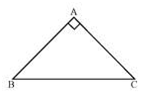It is given that

AB = AC

C = B (Angles opposite to equal sides are also equal)

In ΔABC,

A + B + C = 180º (Angle sum property of a triangle)

90º + B + C = 180º

90º + B + B = 180º

2 B = 90º

B = 45º

B = C = 45º

Question 8 :

Show that the angles of an equilateraltriangle are 60º each.

Answer 8 :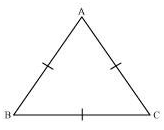Let us consider that ABC is an equilateral triangle.

Therefore, AB = BC = AC

AB = AC

C = B (Angles opposite to equal sides of a triangle are equal)

Also,

AC = BC

B = A (Angles opposite to equal sides of a triangle are equal)

Therefore, we obtain

A = B = C

In ΔABC,

A + B + C = 180°

A + A + A = 180°

3A = 180°

A = 60°

A = B = C = 60°

Hence, in an equilateral triangle, all interior anglesare of measure 60º

Todays Deals### Chapter 7 Triangles Ex-7.2 Contributorskrishan

Name:
Email:

# Latest News# 9000 interview questions in different categories